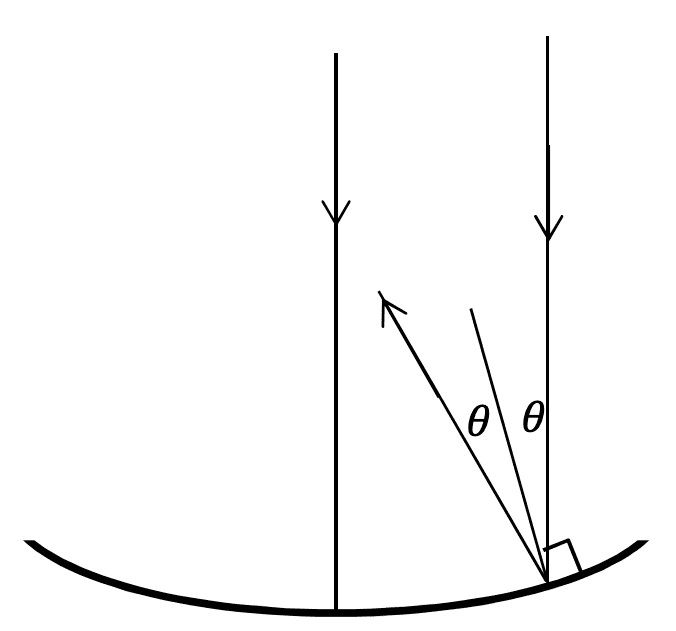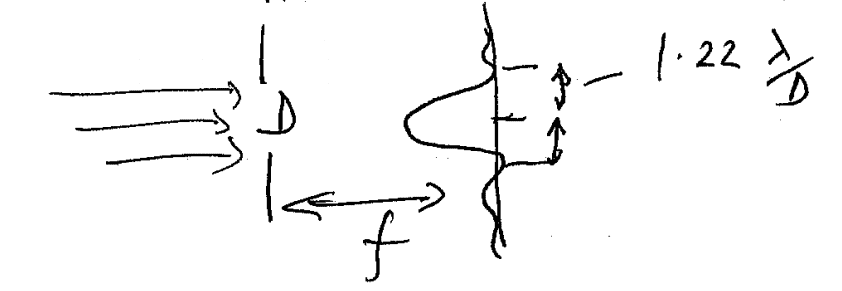# Drop in intensity when light is focused to a point (telescope mirror)

The parabolic mirror pictured below is such that all incident rays, neglecting diffraction, are reflected towards a focus.A question states that the light cannot be focused to a point precisely, since there exists a circular diffraction pattern in the focal plane. The following diagram is given:I'm trying to understand how to calculate the drop in intensity at the image, supposing the intensity of light from the source before it enters the telescope is known. I understand that we can approximate to a reasonable degree of accuracy that only the light in the central maximum within ##\theta = \frac{1.22\lambda}{2}## need be considered; then a ratio of areas can be used for the intensity.

But I can't actually see what ##D## is referring to in the second figure; I assume ##f## is the focal length. Where is the diffraction actually occurring in the parabolic mirror? Thanks!

Last edited by a moderator:

2022 Award
D is the primary mirror diameter - the second diagram has "unfolded" the optical path at the mirror, so the mirror is just a hole. Note that this is implicitly assuming that you're trying to image a point source with a circular primary mirror and a negligible secondary - life is more complicated in reality!

•etotheipi
Gold Member
The parabolic mirror pictured below is such that all incident rays, neglecting diffraction, are reflected towards a focus.

View attachment 255765

A question states that the light cannot be focused to a point precisely, since there exists a circular diffraction pattern in the focal plane. The following diagram is given:

View attachment 255766

I'm trying to understand how to calculate the drop in intensity at the image, supposing the intensity of light from the source before it enters the telescope is known. I understand that we can approximate to a reasonable degree of accuracy that only the light in the central maximum within ##\theta = \frac{1.22\lambda}{2}## need be considered; then a ratio of areas can be used for the intensity.

But I can't actually see what ##D## is referring to in the second figure; I assume ##f## is the focal length. Where is the diffraction actually occurring in the parabolic mirror? Thanks!
Traditionally we would say that the aperture is composed of Huygens's Sources and these produce the pattern you see in the vicinity of the focus. It is like a diffraction grating.
Conversely, for a searchlight or dish antenna, if we place a point source at the focus, the searchlight beam will have a finite angular width and will have side lobes in a similar way.

•etotheipi
Homework Helper
2022 Award
•etotheipi
Homework Helper
Gold Member
Last edited:
•etotheipi
Homework Helper
Gold Member
To add to the above, there are two ways to compute the diffraction pattern result from an aperture plus a focusing optic:
1) You can simply treat the aperture with (far-field) diffraction theory and assume that the optical element gives you the far-field diffraction pattern (from the aperture) in the focal plane, (with a conversion factor ## x=f \theta ##),
or
2) you can assume the Huygens sources are located on the focusing optic, with a phase determined by the shape of the focusing optic, and you compute the near-field diffraction pattern in the focal plane. For the paraboloid, ##4fz=r^2 ## gives the shape of the paraboloid as a function of ## r ##, and ## \Delta=2z =r^2/(2f)## is how much the optical path length is shortened as a function of ## r ##, from which the phases of the Huygens sources can be computed.

Last edited:
•etotheipi
You might find this Insights article that I wrote on the topic of interest: https://www.physicsforums.com/insights/diffraction-limited-spot-size-perfect-focusing/
also see https://www.physicsforums.com/threa...erfect-focusing-comments.907511/#post-5749159 post 13 includes a link to a homework problem on the topic.

Thank you, this is a really useful resource.

I had a few (probably really silly) questions that I was hoping you could clarify; for the relations like ##A_{f} = (\Delta x)^{2}##, are the factors of ##\pi## left out because it's only a constant of proportionality and we can use ratios most of the time? And also, for ##\Delta x = \frac{\lambda f}{b}##, why do we not need the factor of 1.22?

Lastly it's mentioned that ##e_{f} \propto A##; is this because the number of incident rays is proportional to the area, so the number of rays interfering at the focus and consequently the resultant amplitude when they all superpose is also proportional to ##A##?

Last edited by a moderator:
•Homework Helper
Gold Member
I had a few (probably really silly) questions that I was hoping you could clarify; for the relations like Af=(Δx)2Af=(Δx)2A_{f} = (\Delta x)^{2}, are the factors of ππ\pi left out because it's only a constant of proportionality and we can use ratios most of the time? And also, for Δx=λfbΔx=λfb\Delta x = \frac{\lambda f}{b}, why do we not need the factor of 1.22?
In the Insights article, I believe the formula I have for the focused intensity is exact. There then is the calculation (estimate) for spot size area, and perhaps the best estimate is one for effective area ##A_{effective} ## so that ## P=E_{incident} A_{aperture}=E_{focused}A_{effective} ##. What is often presented in the literature for an estimated spot size is to use the location ##r ## of the first zero in intensity of the Airy disc.(see also the homework problem that I previously linked in post 13: https://www.physicsforums.com/threa...n-using-bessel-functions.916241/#post-5775639 see especially post 9 of this homework problem.
In the Insights article, I used a square aperture, (rather than circular), where the diffraction integrals are more readily computed, but the principles are similar. ## \\ ## For the focused intensity being proportional to second power of the aperture area, you got the right idea=since the path distances of each of the rays to the focal point are all identical, there should and will be complete constructive interference, with resultant ## e_f ## proportional to the aperture area, making the energy intensity ##E_{focused} ## proportional to the second power of the aperture area. Here once again, ## E ## is watts/m^2, and not an electric field amplitude, for which I am using little ## e ##.

Last edited:
•etotheipi
Homework Helper
Gold Member
One thing that may be worth mentioning to make sure it doesn't get lost in the mathematics=I stated it in the Insights article, and qualitatively, it is a very important feature: The focused intensity is proportional to the second power of the aperture area, while the focused spot size shrinks when you increase the aperture (focusing optic) area. If you use a focusing optic with a small diameter, the resulting focused spot size can be rather large. If you want a small focused spot, it requires a lens with a somewhat large diameter, i.e. relative to the focal length.

•etotheipi